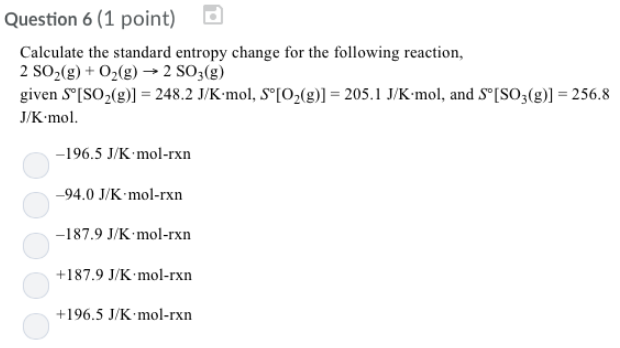Chemistry Practice Problems Entropy Practice Problems Solution: Calculate the standard entropy change for the foll...

🤓 Based on our data, we think this question is relevant for Professor Hernandez's class at UCF.

# Solution: Calculate the standard entropy change for the following reaction, 2SO2 (g) + O2 (g) → 2SO3 (g) given S° [SO2 (g)] = 248.2 J/K • mol. S° [O2 (g)] = 205. 1 J/K • mol, and S° [SO3 (g)] = 256.8 J/K • mol. (a) -196.5 J/K • mol-rxn (b) -94.0 J/K • mol-rxn (c) -187.9 J/K • mol-rxn (d) +187.9 J/K • mol-rxn (e) +196.5 J/K • mol-rxn

###### Problem

Calculate the standard entropy change for the following reaction,

2SO2 (g) + O2 (g) → 2SO3 (g)

given S° [SO2 (g)] = 248.2 J/K • mol. S° [O2 (g)] = 205. 1 J/K • mol, and S° [SO3 (g)] = 256.8 J/K • mol.

(a) -196.5 J/K • mol-rxn

(b) -94.0 J/K • mol-rxn

(c) -187.9 J/K • mol-rxn

(d) +187.9 J/K • mol-rxn

(e) +196.5 J/K • mol-rxnView Complete Written Solution

Entropy

Entropy

#### Q. Entropy of Reaction for Nitrogen Dioxide Formation Nitric oxides. NO and NO2, contribute to air pollution, acid rain, and the depletion of the ozone l...

Solved • Mon Sep 03 2018 13:20:25 GMT-0400 (EDT)

Entropy

#### Q. Part BCalculate the standard entropy change for the reaction P4 (g) + 5O2 (g) → P4O10 (s) using the data from the following table: Express your answer...

Solved • Mon Sep 03 2018 13:16:28 GMT-0400 (EDT)

Entropy

#### Q. Calculate the standard entropy change for the following reaction at 25°C. S° values can be found here. Mg(OH)2 (s) + 2HCl (g) → MgCl2 (s) + 2H2O (g)

Solved • Wed Aug 29 2018 12:43:31 GMT-0400 (EDT)

Entropy

#### Q. The ΔHvap of a certain compound is 28.26 kJ mol and its ΔSvap is 50.01 J•mol-1•K-1. What is the boiling point of this compound?

Solved • Tue Aug 28 2018 12:51:36 GMT-0400 (EDT)